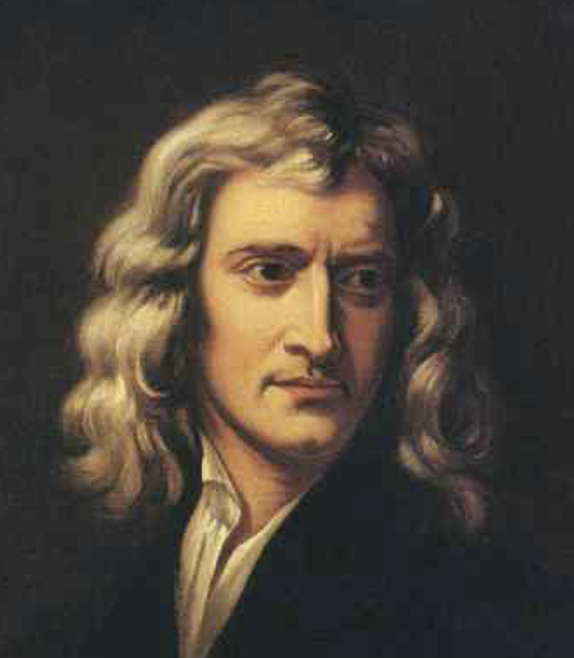# TL;DR Science: The Concept of MassBy Philip Antonopoulos
October 07, 2022 · 4 minute read

Physics### Introduction

Mass is a concept that we all know from our everyday lives. It describes a quantity and is used for practical reasons in commerce. Depending on the country people have an intuitive notion of what a unit of mass describes. For example, in Europe where the kilogram is widely used, people intuitively know that 6 oranges approximately weigh a kilogram. But what does the phrase ‘weigh a kilogram’ mean?

### Gravity and Mass

Weight and mass are two terms used interchangeably in everyday life. This is because the way we usually measure mass is with a scale thereby its weight. But weight is not mass; it is a force that has direction and magnitude and makes objects accelerate downwards. The confusion between weight and mass arises from the relationship that exists between what we call the gravitational mass (which corresponds to a quantity that describes fields in physics such as the electric field) and the gravitational force i.e. weight. This relationship is summarized in the equation W = mg, where g is the gravitational constant and W m are respectively the gravitational force and mass. The essence of this equation, though, is that the force of gravity i.e. weight is directly proportional to the gravitational mass of an object. In other words, if an object has more mass the more it will be pulled toward the center of the earth. But this is not the only place where mass appears in physics…### Newton and Mass

In high school, we learn about Newton’s laws of gravitation and motion. The previous section focused on Newton’s gravitational laws. But Newton’s laws of motion include the concept of mass as well. Specifically, Newton’s second law F = ma describes the relationship between force (how strongly you push something) and acceleration (how quickly something changes speed (in most cases from rest). In other words, when you push something harder it will accelerate quicker, but how much quicker is dictated by the mass of the said object. This is what is called inertial mass. Breaking from our empirical norms this mass does not describe how much ‘stuff’ is contained in an object; rather it describes its inertia (how difficult it is to move the said object). As such, you don’t need the presence of a gravitational field for this mass to exist. You could perform calculations considering the object was in outer space (as is done in most introductory physics classes) and the law would still apply.### Reconciling Gravitational and Inertial Mass

These two masses need not be related as they describe two unrelated situations. But interestingly, they are equal. This can be measured by any simple experiment and can be empirically understood. But this makes it far from a trivial observation. Conversely, one of the axioms (statements that hold without proof by empirical experience) of the most successful physics theory ever devised, General Relativity, is the Equivalence Principle which states that the said masses are equivalent.This conclusion is far from the most interesting thing about the concept of mass. Mass appears in other theories such as Special Relativity where it is different according to your frame of reference (a bizarre and counter-intuitive finding). Additionally, mass appears in one of the most famous physics equations which describes how mass is energy in disguise. Finally, I’d like to add that mass is still the subject of ongoing physics research, most famously in the discovery of the Higgs boson which had to do with the search for the origin of mass.

### TL;DR

The concept of mass in our everyday life appears to be very intuitive. But when studying most physical phenomena you find out that it is quite an abstract and mysterious concept to grasp. Specifically, it appears in a whole sort of unrelated physics phenomena. In fact, I would argue that a great number of physics breakthroughs include the concept of mass in some way: from Newton’s laws of motion and gravity; to Einstein’s special and general relativity; to modern-day particle physics with the discovery of the Higgs boson.

References

2.  Serway, Raymond A. Physics for Scientists & Engineers with Modern Physics. Philadelphia: Saunders College Pub., 19861983